Get inspired by the success stories of our students in IIT JAM MS, ISI  MStat, CMI MSc DS.  Learn More

# CLT and Confidence Limits | ISI MStat 2016 PSB Problem 8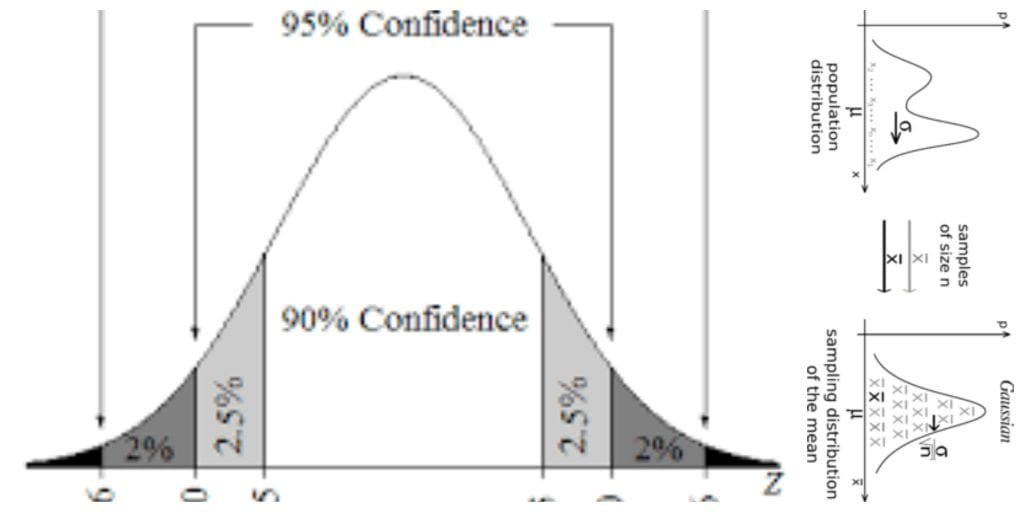This is a problem from ISI MStat Examination 2016. This primarily tests the student's knowledge in finding confidence intervals and using the Central Limit Theorem as a useful approximation tool.

## The Problem:

Let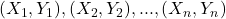be independent and identically distributed pairs of random variables with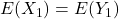,, and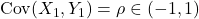(a) Show that there exists a function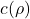such that :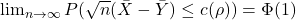, where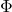is the cdf of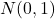(b)Given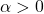, obtain a statistic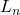which is a function ofsuch that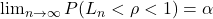.

## Prerequisites:

(a)The Central Limit Theorem and Convergence of Sequence of RVs

(b)Idea of pivots and how to obtain confidence intervals.

## Solution:

(a) See that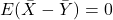.

See that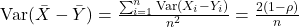Take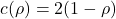.

By the Central Limit Theorem,

see that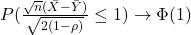as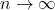(b) Let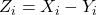's are iid with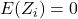and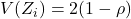Again, by CLT,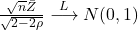Use this as the pivot to obtain an asymptotic confidence interval for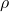.

See that, where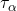: upper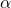point of.

Equivalently , you can write,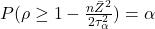, as.

Thus ,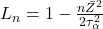.

## Food For Thought:

Suppose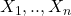are equi-correlated with correlation coefficient.

Given,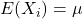,, for.

Can you see that?

It's pretty easy right? Yeah, I know 😛 . I am definitely looking forward to post inequalities more often .

This may look trivial but it is a very important result which can be used in problems where you need a non-trivial lower bound for.

Well,well suppose now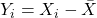.

Can you show that a necessary and sufficient condition for,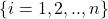to have equal variance is that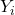should be uncorrelated with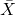?

Till then Bye!

Stay Safe.

This is a problem from ISI MStat Examination 2016. This primarily tests the student's knowledge in finding confidence intervals and using the Central Limit Theorem as a useful approximation tool.

## The Problem:

Letbe independent and identically distributed pairs of random variables with,, and(a) Show that there exists a functionsuch that :, whereis the cdf of(b)Given, obtain a statisticwhich is a function ofsuch that.

## Prerequisites:

(a)The Central Limit Theorem and Convergence of Sequence of RVs

(b)Idea of pivots and how to obtain confidence intervals.

## Solution:

(a) See that.

See thatTake.

By the Central Limit Theorem,

see thatas(b) Let's are iid withandAgain, by CLT,Use this as the pivot to obtain an asymptotic confidence interval for.

See that, where: upperpoint of.

Equivalently , you can write,, as.

Thus ,.

## Food For Thought:

Supposeare equi-correlated with correlation coefficient.

Given,,, for.

Can you see that?

It's pretty easy right? Yeah, I know 😛 . I am definitely looking forward to post inequalities more often .

This may look trivial but it is a very important result which can be used in problems where you need a non-trivial lower bound for.

Well,well suppose now.

Can you show that a necessary and sufficient condition for,to have equal variance is thatshould be uncorrelated with?

Till then Bye!

Stay Safe.

This site uses Akismet to reduce spam. Learn how your comment data is processed.

### Knowledge Partner